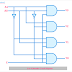## What are Decoders?

The decoders are combinational digital electronic circuits that decode or help to detect binary signals or particular codes in the form of 0 or 1. Generally, the decoders convert or decode the n input lines into a maximum of 2n output lines. The decoder is basically an electronic circuit and its main building blocks are logic gates that are made of transistors. The decoder digital device comes in form of an integrated circuit and it is used in so many useful applications. In this article, we are going to know all the basics of a decoder.

## Types of Decoders

According to the input and output lines the main three types of decoders are,
1. 2 to 4 Decoder
2. 3 to 8 Decoder
3. 4 to 16 Decoder
2 to 4 decoder means it has two input lines and 4 output lines. Same as the 3 to 8 decoder has three inputs and 8 output lines and the 4 to 16 decoder has four input and 16 output lines.

## Decoder Circuit Diagram

Here, you can see the circuit diagram of the 2 to 4 decoder.

You can see the 2 to 4 decoder circuit is built with logic AND gates and logic NOT Gates. The AND gates are having three input terminals. One terminal of each of them is connected together and brought out as the Enable Terminal. The input terminals are named as A0 and A1 whereas the output terminals are named as Y0, Y1, Y2, Y3, etc.

## Decoder Block Diagram

Here, you can see the block diagram of decoder. The block diagram of decoder helps to find out and understand their inputs and outputs.

2 to 4 Decoder Block Diagram

3 to 8 Decoder Block Diagram

You can see in the above block diagram, the 3 to 8 decoder can be made by connecting two 2 to 4 decoders. Each of the 2 to 4 decoders are had two input terminals and four output terminals so the both two input terminals are connected in parallel and they are named as A0 and A1. On the other hand, Enable terminal of the both decoders is connected through a not gate and it is named as A3. So a total of three input terminals are formed. The total of four output terminals of each decoder builds a total of eight terminals.

4 to 16 Decoder Block Diagram

You can see in the above block diagram that we can build a 4 to 16 decoder using two 3 to 8 decoders. Each of the 3 to 8 decoders has three input terminals and 8 output terminals. Now the three input terminals are connected in parallel and named as A0, A1, A2. The enable terminal of each decoder is connected through a not gate and it is named as A3. The total of eight terminals of each decoder build a total of 16 output terminals of the circuit.

## Decoder Truth Table

Here, you can see the truth table of 2 to 4 decoder circuits. Here, the Enable input is E, data inputs are A1 and A0, and the outputs are Y3, Y2, Y1, and Y0

The formula or equation for the boolean functions of each output are,

Y3 = E.A1.A0

Y2 = E.A1.A0'

Y1 = E.A1'.A0

Y0 = E.A1'.A0'

What are Decoders? Block Diagram, Truth Table, TypesReviewed by Author on February 11, 2022 Rating: 5